# O KINETICS AND EQUILIBRIUM Writing the pressure equilibrium expression for a heterogeneous equilibrium Write the pressure...O KINETICS AND EQUILIBRIUM Writing the pressure equilibrium expression for a heterogeneous equilibrium Write the pressure equilibrium constant expression for this reaction. CaO(s)+CO2(g) +CaCO3(s) x S ?

explanation:

we write the equillbria-

CaO(s) + CO2(g) <=====> CaCO3 (s),

Let us consider,

p[CaO] = partial pressure of CaO

p[CO2] = partial pressure of CO2.

p[CaCO3] = partial pressure of CaCO3.

Then pressure equillbirium constant expression ,Kp can be written as:

Kp = p[CaO(s)]*p[CO2(g)]/p[CaCO3 (s)]

Since , activities of solids and liquids are unity ,and then the partial pressure should also be omitted from above expression, therefore,

Kp = 1/[CO2 (g)]

#### Earn Coin

Coins can be redeemed for fabulous gifts.

Similar Homework Help Questions
• ### O KINETICS AND EQUILIBRIUM Writing the pressure equilibrium expression for a heterogeneous equilibrium d o Write...O KINETICS AND EQUILIBRIUM Writing the pressure equilibrium expression for a heterogeneous equilibrium d o Write the pressure equilibrium constant expression for this reaction. 2 HgO(s)- 2 Hg(0)+ O2(9) x 5 ?

• ### O KINETICS AND EQUILIBRIUM Writing the pressure equilibrium expression for a heterogeneous equilibrium Write the pressure...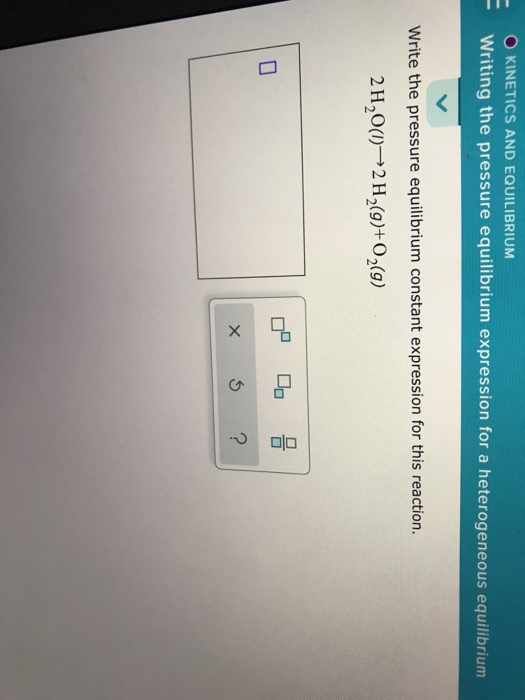O KINETICS AND EQUILIBRIUM Writing the pressure equilibrium expression for a heterogeneous equilibrium Write the pressure equilibrium constant expression for this reaction. 2H,0(1)——2 H2(9)+02(9) x 5 ?

• ### - O KINETICS AND EQUILIBRIUM Writing the concentration equilibrium expression for a heterogeneous equil... G Write...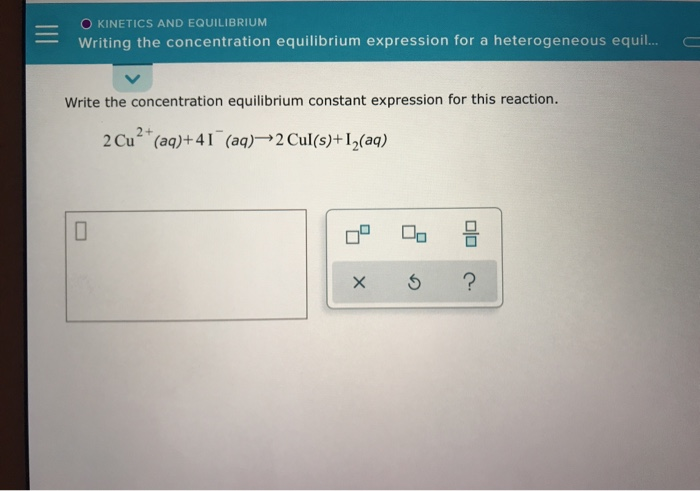- O KINETICS AND EQUILIBRIUM Writing the concentration equilibrium expression for a heterogeneous equil... G Write the concentration equilibrium constant expression for this reaction. 2 Cu?+ (aq)+41 (aq) 2 Cul(s)+12(aq) x 5 ?

• ### - - O KINETICS AND EQUILIBRIUM Writing the concentration equilibrium expression for a heterogeneous equil... a...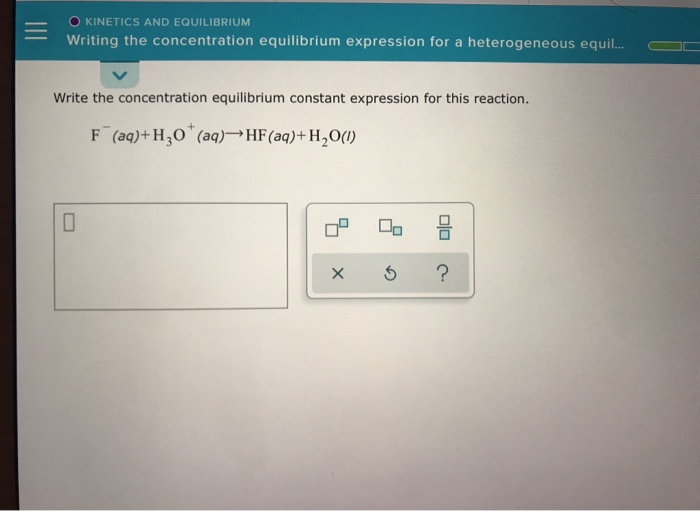- - O KINETICS AND EQUILIBRIUM Writing the concentration equilibrium expression for a heterogeneous equil... a Write the concentration equilibrium constant expression for this reaction. F (aq)+H30* (aq)—HF (aq)+H20(1) x ?

• ### O KINETICS AND EQUILIBRIUM Writing the concentration equilibrium expression for a heterogeneous equil... O - O...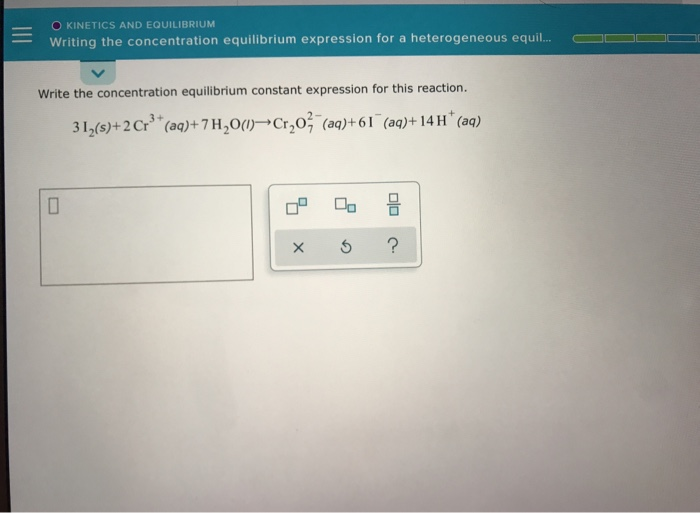O KINETICS AND EQUILIBRIUM Writing the concentration equilibrium expression for a heterogeneous equil... O - O O O Write the concentration equilibrium constant expression for this reaction. 312(\$)+2 Crº* (aq)+7H20(1) Cr,03(aq)+61 (aq)+ 14 H+ (aq) x ?

• ### - - O KINETICS AND EQUILIBRIUM Writing the concentration equilibrium expression for a heterogeneous equil... O...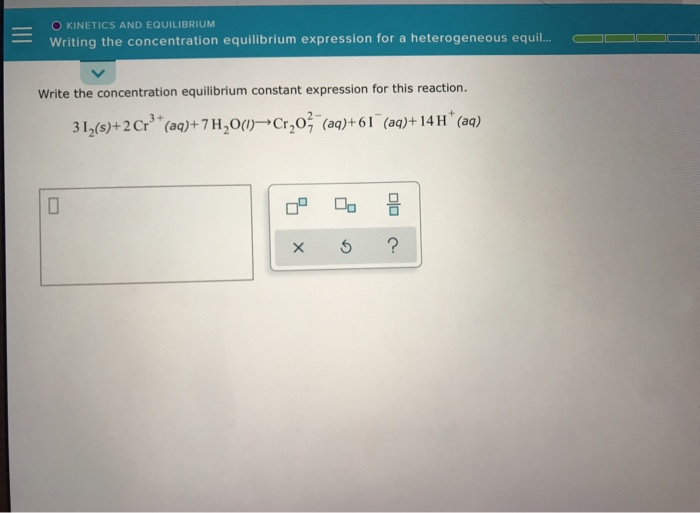- - O KINETICS AND EQUILIBRIUM Writing the concentration equilibrium expression for a heterogeneous equil... O O O Write the concentration equilibrium constant expression for this reaction. 31,(\$)+2 Crº* (aq)+7H20(1) Cr,03(aq)+61 (aq)+14 H+ (aq) xs ?

• ### - -us-east-1-prod-Fleet01-X... Ver Capitulo 184 Kara Sevda O O KINETICS AND EQUILIBRIUM Writing the pressure equilibrium...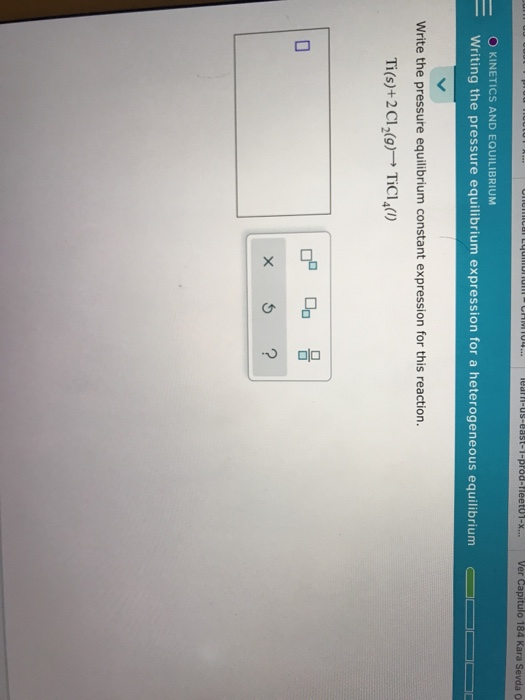- -us-east-1-prod-Fleet01-X... Ver Capitulo 184 Kara Sevda O O KINETICS AND EQUILIBRIUM Writing the pressure equilibrium expression for a heterogeneous equilibrium D O O Write the pressure equilibrium constant expression for this reaction. Ti(s)+2 C12(9) TiCl (0) x 5 ?

• ### O KINETICS AND EQUILIBRIUM Writing a pressure equilibrium constant expression Sulfur trioxide decomposes to form sulfur...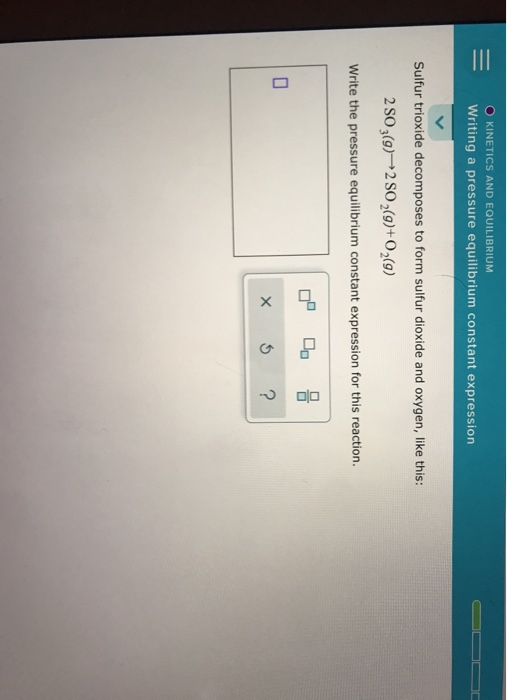O KINETICS AND EQUILIBRIUM Writing a pressure equilibrium constant expression Sulfur trioxide decomposes to form sulfur dioxide and oxygen, like this: 2 SO 3(9)+2 SO 2(9)+02(9) Write the pressure equilibrium constant expression for this reaction. X ?

• ### O KINETICS AND EQUILIBRIUM Writing a pressure equilibrium constant expression Phosphorus tribromide decomposes to form phosphorus...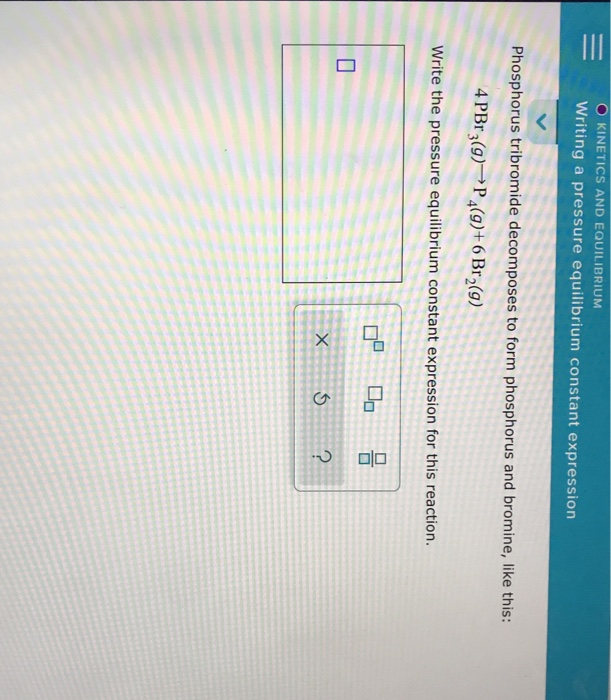O KINETICS AND EQUILIBRIUM Writing a pressure equilibrium constant expression Phosphorus tribromide decomposes to form phosphorus and bromine, like this: 4 PBr 3(9)–P4(9)+6 Br2(9) Write the pressure equilibrium constant expression for this reaction. .

• ### 25. Write the equilibrium constant expression for the following chemical reaction: CaCO3(s) -----------> CaO(s) + CO2(g)...25. Write the equilibrium constant expression for the following chemical reaction: CaCO3(s) -----------> CaO(s) + CO2(g) < O K=[CO2) - K= [CO2][CaO/CaCO3] OK= 1/(CO2) K=[Ca] 24. Consider the following chemical reaction: Ba(OH)2(aq) + H3PO4 (aq) > Baz(PO4)3 (s) + H2O(1) 20.00 mL. of 0.200 M of phosphoric acid reacts with 18.35 ml.of barium hydroxide solution. Calculate molarity of barium hydroxide solution. 4.32M 0.327 M 0.0211M 1.8E M